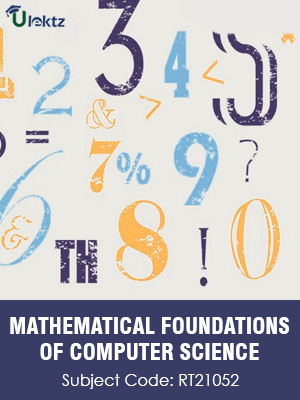uLektz apps

•My WalletMy Order
•My Profile
•My Connections
•My Books
•My Videos
•My Tests
•My Calender
•My Messages
•My Shopping Cart
•My Orders
•Account Settings
•Help

uLektz apps

Book DetailsMATHEMATICAL FOUNDATIONS OF COMPUTER SCIENCE

Course Code:RT21052

Author:uLektz

University:

Regulation:2013

Categories:Computer Science

Format :ePUB3 (DRM Protected)

Type :eBook

FREE

Description :MATHEMATICAL FOUNDATIONS OF COMPUTER SCIENCE of RT21052 covers the latest syllabus prescribed by JNTU Kakinada for regulation 2013. Author: uLektz, Published by uLektz Learning Solutions Private Limited.

Note : No printed book. Only ebook. Access eBook using uLektz apps for Android, iOS and Windows Desktop PC.

Topics
Unit I: Mathematical Logic :

1.1 Propositional Calculus: Statements and Notations - Connectives - Truth Tables

1.2 Tautologies - Equivalence of Formulas, Duality law - Tautological Implications

1.3 Normal Forms - Theory of Inference for Statement Calculus

1.4 Consistency of Premises - Indirect Method of Proof

1.5 Predicate calculus: Predicative Logic

1.6 Statement Functions, Variables and Quantifiers - Free & Bound Variables - Inference theory for predicate calculus

Unit II : Number Theory & Induction:

2.1 Properties of integers - Division Theorem - The Greatest Common Divisor

2.2 Euclidean Algorithm - Least Common Multiple - Testing for Prime Numbers

2.3 The Fundamental Theorem of Arithmetic - Modular Arithmetic (Fermat’s Theorem and Euler ‘s Theorem) - Mathematical Induction: Principle of Mathematical Induction, exercises

Unit III: Set Theory:

3.1 Introduction, Operations on Binary Sets

3.2 Principle of Inclusion and Exclusion

3.3 Relations: Properties of Binary Relations - Relation Matrix and Digraph - Operations on Relations, Partition and Covering

3.4 Transitive Closure, Equivalence, Compatibility - Equivalence - Compatibility

3.5 Partial Ordering Relations - Hasse Diagrams

3.6 Functions: Bijective Functions - Composition of Functions

3.7 Inverse Functions, Permutation Functions - Permutation Functions

3.8 Recursive Functions

Unit IV: Graph Theory:

4.1 Basic Concepts of Graphs

4.2 Sub graphs

4.3 Matrix Representation of Graphs: Adjacency Matrices - Incidence Matrices

4.4 Isomorphic Graphs

4.5 Paths and Circuits - Eulerian and Hamiltonian Graphs

4.6 Multigraphs, (Problems and Theorems without proofs) - Planar Graphs

4.7 Euler’s Formula

4.8 Graph Colouring and Covering

4.9 Chromatic Number ( Problems and Theorems without proofs)

4.10 Trees - Directed trees - Binary Trees - Decision Trees

4.11 Spanning Trees: Properties - Algorithms for Spanning trees and Minimum Spanning Tree

Unit V: Algebraic Structures:

5.1 Algebraic Structures: Lattice: Properties - Lattices as Algebraic Systems

5.2 Algebraic Systems with one Binary Operation - Properties of Binary operations

5.3 Semi groups and Monoids - Homomorphism of Semi groups and Monoids

5.4 Groups: Abelian Group - Cosets, Subgroups (Definitions and Examples of all Structures) - Algebraic Systems with two Binary Operations: Rings

5.5 Combinatorics: Basic of Counting - Permutations, Combinations

5.6 Derangements - Permutations with Repetition of Objects - Circular Permutations - Restricted Permutations - Restricted Combinations - Pigeonhole Principle and its Application

5.7 Binomial Theorem: Binomial and Multinomial Coefficients

5.8 Generating Functions of Permutations and Combinations

5.9 The Principles of Inclusion – Exclusion

Unit VI: Recurrence Relation:

6.2 Partial Fractions - Calculating Coefficient of Generating Functions Recurrence Relations

6.3 Formulation as Recurrence Relations - Solving linear homogeneous recurrence Relations by substitution

6.4 Generating functions and The Method of Characteristic Roots - Solving Inhomogeneous Recurrence Relations

Related Books